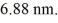# [Solved] An Electron Is Trapped in an Infinite Square Well (A

Question 17
Multiple Choice

## An electron is trapped in an infinite square well (a box) of widthFind the wavelength of photons emitted when the electron drops from the n = 5 state to the n = 1 state in this system. (c = 3.00 × 108 m/s, h = 6.626 × 10-34J ∙ s, mel = 9.11 × 10-31 kg)

A) 6.49 μm
B) 5.45 μm
C) 5.91 μm
D) 7.07 μm

10+ million students use Quizplus to study and prepare for their homework, quizzes and exams through 20m+ questions in 300k quizzes.

### Physics & Astronomy

Explore our library and get Introduction to Physical Science Homework Help with various study sets and a huge amount of quizzes and questions

120

Study sets

3.1K

Quizzes

233.2K

Questions

Upload material to get free accessInvite a friend and get free accessSubscribe and get an instant access